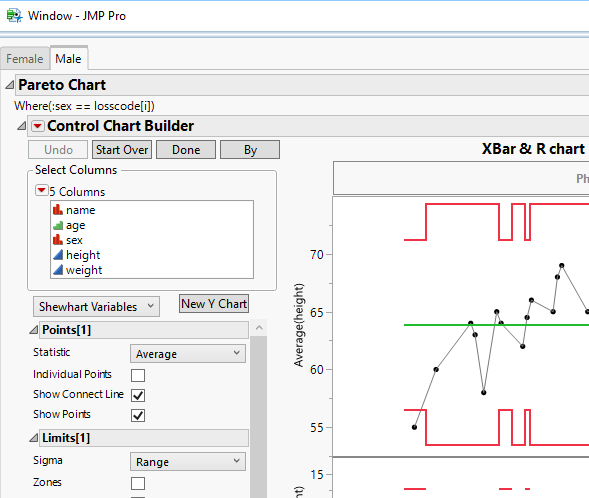Choose Language Hide Translation Bar
Highlighted

## set title for control chart open in mulitple tab

Hi all ,

I would like to change the title of each of my individual  control chart opened in various tab , i have tried set title  ("xxx") but it apprears error , anyone can help ?

``````For( i = 1, i <= n Items(losscode), i++,
losscode[i],ob1 = Outline Box( "Pareto Chart",
dt << Control Chart Builder(
Show Capability( 0 ),
Variables( Subgroup( :weight ), Y( :height ) ),
Chart( Position( 1 ), Limits( Sigma ) ),
Chart( Position( 2 ), Limits( Sigma ) ),
Where( :name == losscode[i] ))

)

)

);``````
2 ACCEPTED SOLUTIONS

Accepted Solutions
Highlighted

## Re: set title for control chart open in mulitple tab

You are very close to getting the syntax correct.  However, if you look at the Tree Structure of the output from the Tab Box() you are creating, you will see that for each tab within the Tab Box() there is a Tab Page Box().  It is within that object that you send the message to change the TitleHere

I suggest that you read up on Tree Structures in the Scripting Guide

Help==>Books==>Scripting Guide

Here is a sample script

``````names default to here(1);
dt=open("\$SAMPLE_DATA/big class.jmp");

summarize(dt,losscode=by(:sex));

nw=new window("Window", tb=tab box());

For( i = 1, i <= N Items( losscode ), i++,
losscode[i],
ob1 = Outline Box( "Pareto Chart",
dt << Control Chart Builder(
Show Capability( 0 ),
Variables( Subgroup( :weight ), Y( :height ) ),
Chart( Position( 1 ), Limits( Sigma ) ),
Chart( Position( 2 ), Limits( Sigma ) ),
Where( :sex == losscode[i] )
)
)
)
);
tb[tabpagebox(1)]<<title("Female");
tb[tabpagebox(2)]<<title("Male");``````

Jim
Highlighted

## Re: set title for control chart open in mulitple tab

If you want to change the Control Chart Builder to something else, that is just a simple extension off of my previous response``````names default to here(1);
dt=open("\$SAMPLE_DATA/big class.jmp");

summarize(dt,losscode=by(:sex));

nw=new window("Window", tb=tab box());

For( i = 1, i <= N Items( losscode ), i++,
losscode[i],
ob1 = Outline Box( "Pareto Chart",
dt << Control Chart Builder(
Show Capability( 0 ),
Variables( Subgroup( :weight ), Y( :height ) ),
Chart( Position( 1 ), Limits( Sigma ) ),
Chart( Position( 2 ), Limits( Sigma ) ),
Where( :sex == losscode[i] )
)
)
)
);
tb[tabpagebox(1)][OutlineBox(2)]<< set title("Female");
tb[tabpagebox(2)]["Control Chart Builder"] << set title("Male");``````
Jim
3 REPLIES 3
Highlighted

## Re: set title for control chart open in mulitple tab

You are very close to getting the syntax correct.  However, if you look at the Tree Structure of the output from the Tab Box() you are creating, you will see that for each tab within the Tab Box() there is a Tab Page Box().  It is within that object that you send the message to change the TitleHere

I suggest that you read up on Tree Structures in the Scripting Guide

Help==>Books==>Scripting Guide

Here is a sample script

``````names default to here(1);
dt=open("\$SAMPLE_DATA/big class.jmp");

summarize(dt,losscode=by(:sex));

nw=new window("Window", tb=tab box());

For( i = 1, i <= N Items( losscode ), i++,
losscode[i],
ob1 = Outline Box( "Pareto Chart",
dt << Control Chart Builder(
Show Capability( 0 ),
Variables( Subgroup( :weight ), Y( :height ) ),
Chart( Position( 1 ), Limits( Sigma ) ),
Chart( Position( 2 ), Limits( Sigma ) ),
Where( :sex == losscode[i] )
)
)
)
);
tb[tabpagebox(1)]<<title("Female");
tb[tabpagebox(2)]<<title("Male");``````

Jim
Highlighted

## Re: set title for control chart open in mulitple tab

Ths nelson ,

But i wanted to change the title of the control chart builder inside female tab instead of the female tab .

Highlighted

## Re: set title for control chart open in mulitple tab

If you want to change the Control Chart Builder to something else, that is just a simple extension off of my previous response``````names default to here(1);
dt=open("\$SAMPLE_DATA/big class.jmp");

summarize(dt,losscode=by(:sex));

nw=new window("Window", tb=tab box());

For( i = 1, i <= N Items( losscode ), i++,
losscode[i],
ob1 = Outline Box( "Pareto Chart",
dt << Control Chart Builder(
Show Capability( 0 ),
Variables( Subgroup( :weight ), Y( :height ) ),
Chart( Position( 1 ), Limits( Sigma ) ),
Chart( Position( 2 ), Limits( Sigma ) ),
Where( :sex == losscode[i] )
)
)
)
);
tb[tabpagebox(1)][OutlineBox(2)]<< set title("Female");
tb[tabpagebox(2)]["Control Chart Builder"] << set title("Male");``````
Jim